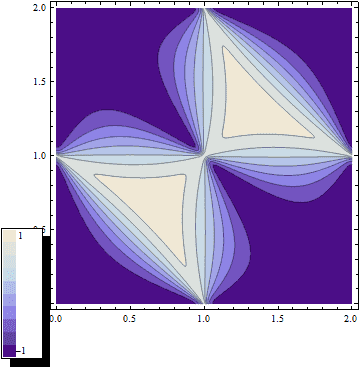# Problem in reporting contour plot mathematica

• Mathematica
• quin

#### quin

Dear friends
I plot a Contour plot from a function with mathematica, but I don't know how can I add a small box near my figure that explain which value refer to which color?

t = 0.01;

m = 0.7820;

ContourPlot[(3 (2 + m t (4 + m t)) - 4 (1 + m t) Cos[\[Pi] x] -
2 Cos[2 \[Pi] x] - 4 (1 + m t) Cos[\[Pi] y] - 2 Cos[2 \[Pi] y] +
4 Cos[\[Pi] (-x + y)] - 4 (1 + m t) Cos[\[Pi] (x + y)] -
2 Cos[2 \[Pi] (x + y)] + 4 Cos[\[Pi] (2 x + y)] +
4 Cos[\[Pi] (x + 2 y)])/(m (6 + m t (6 + m t) -
2 Cos[2 \[Pi] x] - 2 Cos[2 \[Pi] y] -
2 Cos[2 \[Pi] (x + y)])), {x, 0, 2}, {y, 0, 2}]

Reference

Code:
Needs["PlotLegends"]
ShowLegend[
ContourPlot[(3 (2 + m t (4 + m t)) - 4 (1 + m t) Cos[\[Pi] x] -
2 Cos[2 \[Pi] x] - 4 (1 + m t) Cos[\[Pi] y] - 2 Cos[2 \[Pi] y] +
4 Cos[\[Pi] (-x + y)] - 4 (1 + m t) Cos[\[Pi] (x + y)] -
2 Cos[2 \[Pi] (x + y)] + 4 Cos[\[Pi] (2 x + y)] +
4 Cos[\[Pi] (x + 2 y)])/(m (6 + m t (6 + m t) -
2 Cos[2 \[Pi] x] - 2 Cos[2 \[Pi] y] -
2 Cos[2 \[Pi] (x + y)])), {x, 0, 2}, {y, 0,
2}], {ColorData["LakeColors"][1 - #1] &, 10, " 1", "-1"}]`thank you but how you can understand that you must put 1 to -1 for that range? I don't Know how to estimate the range of color data?

thanks alot

Well, I haven't estimated it also, just did it as an example. Do a little research :)

how can I put "PlotLegends -> Automatic" in CountorPlot?
I search google but I couldn't find anything suitable for the above code ! Iwant mathematica to find plotlegend in an automatic way, not by writing for example 1 to -1

thanks

In:= t = 0.01;
m = 0.7820;
ContourPlot[(3 (2 + m t (4 + m t)) - 4 (1 + m t) Cos[\[Pi] x] -
2 Cos[2 \[Pi] x] - 4 (1 + m t) Cos[\[Pi] y] - 2 Cos[2 \[Pi] y] +
4 Cos[\[Pi] (-x + y)] - 4 (1 + m t) Cos[\[Pi] (x + y)] -
2 Cos[2 \[Pi] (x + y)] + 4 Cos[\[Pi] (2 x + y)] +
4 Cos[\[Pi] (x + 2 y)])/(m (6 + m t (6 + m t) -
2 Cos[2 \[Pi] x] - 2 Cos[2 \[Pi] y] -
2 Cos[2 \[Pi] (x + y)])), {x, 0, 2}, {y, 0, 2},
PlotLegends -> Automatic]

Out= ...PlotWithLegendSnipped...

works for me, at least for this example in version 9.

As for being unable to find anything on this, if you Google
Mathematica ContourPlot
the first result is
http://reference.wolfram.com/mathematica/ref/ContourPlot.html
and if you click on Details and Options near the top of that you
see the minimal documentation on the PlotLegends option.
Or you can get the identical information from your Mma help pages.
Values that may be given with Options are unfortunately often
inadequately documented, but this one is better than most.

Last edited:
•1 person
thanks but I have mathematica 7 and when I type that code, it gives error that unknown plotlegends in contourplot ! however, I write : needs"plotlegends'" too.

If anyone is not using the current version of software then it would be really helpful if they made sure to explain that when they ask their question. That will allow people to see if they can think of any workarounds that would be backward compatible with your version when they try to give answers.

What if you use one of the available functions to estimate the minimum and maximum values that your function has inside the plot range you are going to display and use those values to define the upper and lower bounds of your PlotLegends?